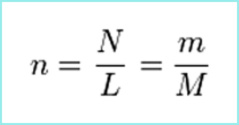Knowledge database: Stoichiometry: Amount of substance Amount of substance (n), as the name itself says, is a measure of the amount of a substance, with the mole being the unit of this physical quantity. One mole is equal to the Avogadro's number of particles. That number, in turn, is equal to the number of atoms in 12 grams of the carbon isotope 12C. Therefore, one mole of any substance has 6.0221415x1023 particles.The amount of substance can simply be calculated by taking the mass of a substance (m) and dividing it by the molar mass of that same substance (M). It can also be obtained by dividing the number of particles (N) by the Avogadro's constant (L) which is numerically equal to the Avogadro's number, but comes with the unit mole-1. Amount of substance is one of the most important physical quantities, not only in chemistry, but in general science, and therefore it is reasonably classified into the seven basic physical quantities of the SI system.